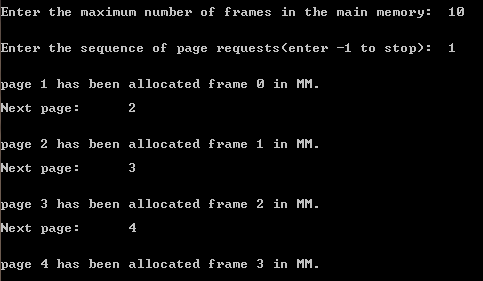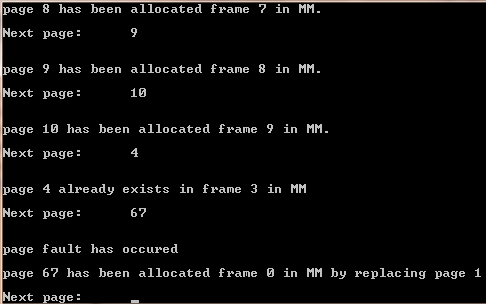# C / C++ Program To Implement LRU Page Replacement Algorithm ( Least Recently Used )

/* In the following C / C++ Program, The LRU (Least Recently Used) algorithm is used, when a page fault occurs, it throw out the page that has been unused for the longest time. This strategy is called LRU (Least Recently Used) paging. To fully implement LRU, it is necessary to maintain a linked list of all pages in memory, with the most recently used page at the front and the least recently used page at the rear. The difficulty is that the list must be updated on every memory reference. Finding a page in the list, deleting it, and then moving it to the front is a very time consuming operation, even in hardware (assuming that such hardware could be built). */
/*The C++ Code Implementation of LRU Page Replacement Algorithm is given below & it is being tested in MS Visual Studio 2010*/

#include<iostream>
#include<stdio.h>
#include<stdlib.h>
#include<ctype.h>

using namespace std;

int mindis(int *dis,int max)
{
int m=0;
for(int i=0;i<max;i++)
{
if(dis[i]<dis[m]) m=i;
}
return(m);
}

int found(int x,int *l,int max)
{
for(int i=0;i<max;i++)
if(l[i]==x){return(i);}
return(-1);
}

int main()
{
cout<<"\n\nEnter the maximum number of frames in the main memory:\t";
int max,x,k=0,j=0,res;
cin>>max;
int *l=new int[max];
int *a=new int;
for(int i=0;i<max;i++)l[i]=-1;
cout<<"\n\nEnter the sequence of page requests(enter -1 to stop):\t";

int *dis=new int[max];
int *flag=new int[max];
for(int i=0;i<max;i++) flag[i]=0;

while(1)
{
cin>>x;
if(x==-1) {cout<<"\n\n";break;}
else{

if(j<max)
{
if((res=found(x,l,max))!=-1) {cout<<"\n\npage "<<x<<" already exists in frame "<<res<<" in MM";
cout<<"\n\nNext page:\t";a[k++]=x;}
else
{
cout<<"\n\npage "<<x<<" has been allocated frame "<<j<<" in MM.";
l[j++]=a[k++]=x;
cout<<"\n\nNext page:\t";
}
}
else
{
if((res=found(x,l,max))!=-1) {cout<<"\n\npage "<<x<<" already exists in frame "<<res<<" in MM";
cout<<"\n\nNext page:\t";a[k++]=x;}
else{

cout<<"\n\npage fault has occured";

for(int z=0;z<max;z++) flag[z]=0;

for(int p=0;p<max;p++)
for(int q=k-1;q>=0;q--)
{
if(l[p]==a[q] && flag[p]==0) {dis[p]=q;flag[p]=1;}
else if(flag[p]!=1) dis[p]=-1000000;
}

int pos=mindis(dis,max);

cout<<"\n\npage "<<x<<" been allocated frame "<<pos<<" in MM by replacing page "<<l[pos];
l[pos]=x;
a[k++]=x;

cout<<"\n\nNext page:\t";
}
}
}

}

delete[] l;
delete[] a;
delete[] dis;
delete[] flag;
system("pause");
return(0);
}

Screenshots:LRU Algorithm ImplementaionLRU Algorithm Implementaion